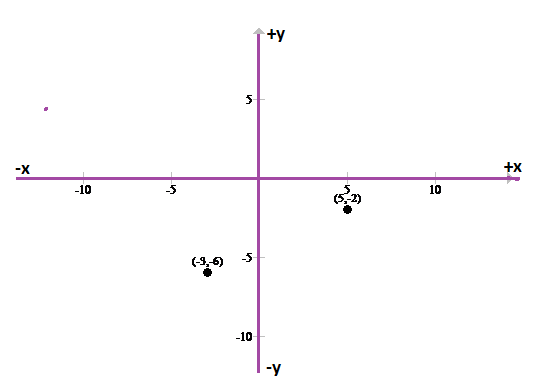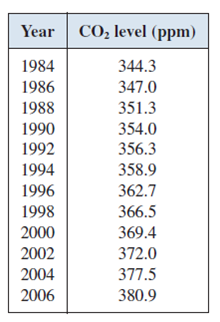# Make a scatter plot of the data.### Precalculus: Mathematics for Calcu...

6th Edition
Stewart + 5 others
Publisher: Cengage Learning
ISBN: 9780840068071### Precalculus: Mathematics for Calcu...

6th Edition
Stewart + 5 others
Publisher: Cengage Learning
ISBN: 9780840068071

#### Solutions

Chapter 1, Problem 4P

a.

To determine

## Make a scatter plot of the data.

Expert Solution

/

### Explanation of Solution

Given information:

The Mauna Loa Observatory, located on the island of Hawaii, has been monitoring carbon dioxide ( CO2 ) levels in the atmosphere since 1958 . The table lists the average annual CO2 levels measured in parts per million (ppm) from 1984 to 2006 .Calculation:

Consider two point P(5,2),Q(3,6) in the coordinate plane,

The scatter plot for points P(5,2),Q(3,6) is as shown,Hence, the function is plotted.

b.

To determine

Expert Solution

y=12x92

### Explanation of Solution

Given information:

The Mauna Loa Observatory, located on the island of Hawaii, has been monitoring carbon dioxide ( CO2 ) levels in the atmosphere since 1958 . The table lists the average annual CO2 levels measured in parts per million (ppm) from 1984 to 2006 .Calculation:

Apply distance formula to find the distance between points P(5,2),Q(3,6) .

PQ=(6(2))2+(35)2=(4)2+(8)2=16+64=80=8.94

Slope of line is ,

m(slope)=6(2)35=6+28=48=12

Now the equation of line will be,

y(2)=12(x5)y+2=12x52y=12x92

Hence, the regression line is y=12x92 .

c.

To determine

Expert Solution

(1,4)

### Explanation of Solution

Given information:

The Mauna Loa Observatory, located on the island of Hawaii, has been monitoring carbon dioxide ( CO2 ) levels in the atmosphere since 1958 . The table lists the average annual CO2 levels measured in parts per million (ppm) from 1984 to 2006 .Calculation:

The mid point of line joining P(5,2),Q(3,6) is,

=5+(3)2,2+(6)2=532,262=22,82=1,4

Hence, the result is, (1,4) .

### Have a homework question?

Subscribe to bartleby learn! Ask subject matter experts 30 homework questions each month. Plus, you’ll have access to millions of step-by-step textbook answers!